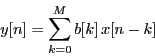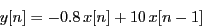# FIR Filters

Finite Impulse Response filters are those filters present a non-zero finite length response when excited with a very brief (ideally an infinite peak) input signal. A linear causal FIR filter can be described by the following difference equationThis operation describe a nonrecursive system, i.e. a system that only depends on current and past samples of the input data stream x[n]

## Creating a FIR filter in the LTPDA

The LTPDA Toolbox allows the implementation of FIR filters by means of the mfir class.

## Creating from a plist

The following example creates an order 64 highpass filter with high frequency gain 2. The filter is designed for 1 Hz sampled data and has a cut-off frequency of 0.2 Hz.

```
pl = plist('type', 'highpass', ...
'order', 64,         ...
'gain',  2.0,       ...
'fs',    1,        ...
'fc',    0.2);
f = mfir(pl)
```

## Creating from a difference equation

The filter can be defined in terms of two vectors specifying the coefficients of the filter and the sampling frequency. The following example creates a FIR filter with sampling frequency 1 Hz and the following recursive equation:```
b  = [-0.8 10];
fs = 1;
f  = mfir(b,fs)
```

## Creating from an Analysis Object

A FIR filter can be generated based on the magnitude of the input Analysis Object or fsdata object. In the following example a fsdata object is first generated and then passed to the mfir constructor to obtain the equivalent FIR filter.

```
fs  = 10;                      % sampling frequency
f   = linspace(0, fs/2, 1000);
y   = 1./(1+(0.1*2*pi*f).^2);  % an arbitrary function
fsd = fsdata(f,y,fs);          % build the fsdata object
f = mfir(ao(fsd));

```

Available methods for this option are: 'frequency-sampling' (uses fir2), 'least-squares' (uses firls) and 'Parks-McClellan' (uses firpm)

## Importing an existing model

The mfir constructor also accepts as an input existing models in different formats:

• LISO files:

```      f = mfir('foo_fir.fil')
```
• XML files:

```      f = mfir('foo_fir.xml')
```
• MAT files:

```      f = mfir('foo_fir.mat')
```
• From repository:

```      f = mfir(plist('hostname', 'localhost', 'database', 'ltpda', 'ID', []))
```